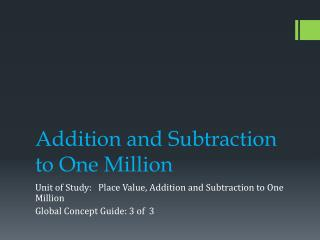DownloadDownload PresentationAddition and Subtraction to One Million

# Addition and Subtraction to One Million

Download Presentation## Addition and Subtraction to One Million

- - - - - - - - - - - - - - - - - - - - - - - - - - - E N D - - - - - - - - - - - - - - - - - - - - - - - - - - -
##### Presentation Transcript

1. Addition and Subtraction to One Million Unit of Study: Place Value, Addition and Subtraction to One Million Global Concept Guide: 3 of 3

2. Content Development While subtracting using the standard algorithm, students must carefully align the digits according to place value. Students must also recognize if the digit being subtracted is greater than the digit it is being subtracted from, they must regroup in order to subtract. Previous work with renaming numbers helps students understand that 3 tens 1 one can be renamed as 2 tens 11 ones. Students continue to use estimation to check the reasonableness of their answers. Since addition and subtraction are inverse operations, students can also use addition to check their answer to a subtraction problem. - Go Math TE 31A

3. Content Development - Go Math TE 35A

4. Refer to the Enrich/Reteach/Intervention slide at the end of this PowerPoint for ideas to differentiate instruction throughout this GCG as needed.